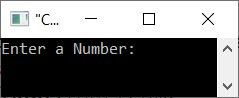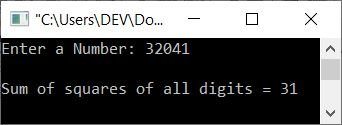# C++ program to find the sum of the squares of the digits of a number

This article provides some programs in C++ that find and print the sum of squares of digits of a given number. The program is created in the following ways:

For example, if the given number is 32041, then the result will be calculated as follows:

```32041 = 32 + 22 + 02 + 42 + 12
= 9 + 4 + 1 + 16 + 1
= 31```

## Using the while loop, compute the sum of squares of a number's digits

The question is, "Write a C++ program that receives a number from the user to find and print the sum of the squares of its digits." The program given below is its answer:

```#include<iostream>

using namespace std;
int main()
{
int num, rem, sq, sum=0;
cout<<"Enter a Number: ";
cin>>num;
while(num>0)
{
rem = num%10;
if(rem==0)
sq = 1;
else
sq = rem*rem;
sum = sum + sq;
num = num/10;
}
cout<<"\nSum of squares of all digits = "<<sum;
cout<<endl;
return 0;
}```

The initial output produced by the above C++ program on finding and printing the sum of squares of digits of a number entered by the user is shown in the snapshot given below:Now supply a number, say 32041, as input and press the ENTER key to find and print the sum of squares of all the digits of 32041 as shown in the snapshot given below:## Using a for loop, find the sum of the squares of a number's digits

This is the last program, created using a for loop, that does the same job as the previous program. Also, this program produces exactly the same output as the previous one.

```#include<iostream>

using namespace std;
int main()
{
int num, rem, sq, sum;
cout<<"Enter a Number: ";
cin>>num;
for(sum=0; num>0; num=num/10)
{
rem = num%10;
if(rem==0)
sq = 1;
else
sq = rem*rem;
sum = sum + sq;
}
cout<<"\nSum of squares of all digits = "<<sum;
cout<<endl;
return 0;
}```

C++ Quiz

« Previous Program Next Program »